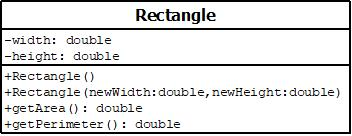# JAVA design a class named Rectangle to represent a rectangle. The class contains: A method named getPerimeter() that returns the perimeter. Two double data fields named width and height that specify...

JAVA

design a class named Rectangle to represent a rectangle. The class contains:

A method named getPerimeter() that returns the perimeter.

• Two double data fields named width and height that specify the width and height of the rectangle. The default values are 1 for both width and height.

• A no-arg constructor that creates a default rectangle.

• A constructor that creates a rectangle with the specified width and height.

• A method named getArea() that returns the area of this rectangle.

• design a class named Rectangle to represent a rectangle. The class contains:

• Two double data fields named width and height that specify the width and height of the rectangle. The default values are 1 for both width and height.

• A no-arg constructor that creates a default rectangle.

• Draw the UML diagram for the class and then implement the class. Write a test program that creates two Rectangle objects-one with width 4 and height 40 and the other with width 3.5 and height 35.9. Display the width, height, area, and perimeter of each rectangle in this order.

• A constructor that creates a rectangle with the specified width and height.

• A method named getArea() that returns the area of this rectangle.

• A method named getPerimeter() that returns the perimeter.

Draw the UML diagram for the class and then implement the class. Write a test program that creates two Rectangle objects-one with width 4 and height 40 and the other with width 3.5 and height 35.9. Display the width, height, area, and perimeter of each rectangle in this order.

public class Exercise09_01
{
public static void main (String[] args)
{
Rectangle rectangle1 = new Rectangle(4,40);

Rectangle rectangle2 = new Rectangle(3.5,3.59);

System.out.println("The details of the first rectangel...");
System.out.println("Width of Rectangle1:" + rectangle1.width);
System.out.println("Height of Rectangle1:" + rectangle1.height);
System.out.println("Area of Rectangle1:" + df.format(rectangle1.getArea()));
System.out.println("Perimeter of Rectangle1:"+ rectangle1.getPerimeter());
System.out.println();

System.out.println("The details of the second rectangel...");
System.out.println("Width of Rectangle2:" + rectangle2.width);
System.out.println("Height of Rectangle2:" + rectangle2.height);
System.out.println("Area of Rectangle2:" + df.format(rectangle2.getArea()));
System.out.println("Perimeter of Rectangle2:"+ rectangle2.getPerimeter());
}
}

public class Rectangle
{
double width = 1.0;
double height = 1.0;
Rectangel() {
}
Rectangel(double newWidth, double newHeight)
{
width = newWidth;
height = newHeight;
}
double getArea()
{
return width * height;
}
double getPerimeter()
{
return 2*(width + height);
}
}

UML Rectangle class digaram-------------------------------*-------------------------------*-------------------------------*
/**Test java program for Rectangle class*/
//Exercise09_01.java
import java.text.DecimalFormat;
public class Exercise09_01
{
public static void main (String[] args)
{
Rectangle rectangle1 = new Rectangle(4,40);
Rectangle rectangle2 = new Rectangle(3.5,3.59);

/**Create an instace of DecimalFormat class*/
DecimalFormat df=new DecimalFormat("#,##.##");

System.out.println("The details of the first rectangel...");
System.out.println("Width of Rectangle1:" + rectangle1.width);
System.out.println("Height of Rectangle1:" + rectangle1.height);
System.out.println("Area of Rectangle1:" + df.format(rectangle1.getArea()));
System.out.println("Perimeter of Rectangle1:"+ rectangle1.getPerimeter());
System.out.println();

System.out.println("The details of the second rectangel...");
System.out.println("Width of Rectangle2:" + rectangle2.width);
System.out.println("Height of Rectangle2:" + rectangle2.height);
System.out.println("Area of Rectangle2:" + df.format(rectangle2.getArea()));
System.out.println("Perimeter of Rectangle2:"+ rectangle2.getPerimeter());
}
}

-------------------------------*-------------------------------*-------------------------------*
//Rectangle.java
public class Rectangle
{
/**Instance variables */
double width ;
double height;
/*Name of the constructor must match with
* the name of the class, Rectangle*/
public Rectangle()
{
width=1;
height=1;
}
/*Name of the constructor must match with
* the name of the class, Rectangle*/
public Rectangle(double newWidth, double newHeight)
{
width = newWidth;
height = newHeight;
}
/**Returns area */
public double getArea()
{
return width * height;
}
/**Returns perimeter */
public double getPerimeter()
{
return 2*(width + height);
}
}/**End of Rectangle class*/

-------------------------------*-------------------------------*-------------------------------*

Sample Output:

The details of the first rectangel...
Width of Rectangle1:4.0
Height of Rectangle1:40.0
Area of Rectangle1:1,60
Perimeter of Rectangle1:88.0

The details of the second rectangel...
Width of Rectangle2:3.5
Height of Rectangle2:3.59
Area of Rectangle2:12.56
Perimeter of Rectangle2:14.18

##### Add Answer of: JAVA design a class named Rectangle to represent a rectangle. The class contains: A method named getPerimeter() that returns the perimeter. Two double data fields named width and height that specify...
More Homework Help Questions Additional questions in this topic.